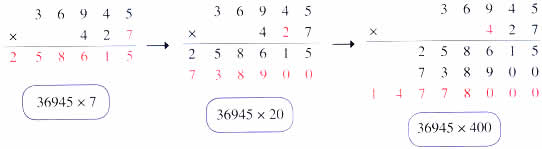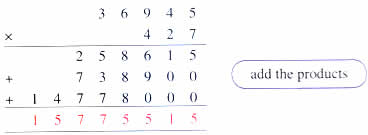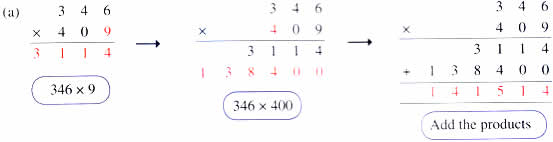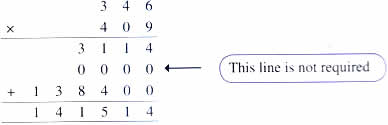Sunday , July 12 2020# NCERT 5th Class (CBSE) Mathematics: The Four Operations And Their Application

## MULTIPLICATION

### Properties of Multiplication:

These are some properties of multiplication that you are familiar with.

1. Any number multiplied by 0 gives zero as the product.
2. Any number multiplied by 1 gives the same number as the product.
3. When the order of the factors is changed the product does not change.

### Multiplying by a 3-Digit Number### Multiplying by Numbers with 0(b)## 10th Class CBSE Mathematics 2018-19

10th Class CBSE Mathematics 2018-19 Time: 3 hours M.M.: 80 Class: 10th Subject: Mathematics Date: 07/03/2019 …